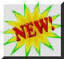Self Study Program,

"Welding Math and Science;" 87 Pages, Part # WMS

This program provides basic information to help welding students and welding supervisors understand welding process characteristics using basic math and science.  Includes and Appendix with Simplified Basic Math Functions and Metric Conversions.

Reading a ruler in US Measurement Units requires working with Fractions.  If the Metric System was being used it would not - more on Metric below.

Rulers and measuring tapes are commonly calibrated in feet and inches.  Instead of segmenting the foot into 10ths of a foot - it is divided into 12 parts called inches.The inch is commonly split into 8, 16 or sometimes 32 parts.  Therefore working with Fractions is a necessity.  A Fraction of an inch is a mathematical way of showing the number of the parts into which it is divided.  For example, 3/8 inches is a measure of 3 of the 8 parts.  The picture left is an example of an inch split into 8 parts or "eighths."  The yellow arrow is pointing to the third line from the start or the 3rd of 8 parts stated as 3/8.  It’s shown with a division line between the numbers since we could divide 3 by 8 and get 0.375 the decimal value.  If we always stated the number of segments measured and the total number of segments into which the inch mark was split, Fractions would be relatively easy.  Unfortunately it is not that easy!  We'll try to make it as painless as possible!The example on the right shows the 4th of the 1/8 inch marks in red.  Since 4 is half  way to 8 - it is the 1/2 inch mark.  We could have said the 4/8 mark which is the same as saying 1/2 .  It is usually desired to display the Fraction by having the lowest whole numbers possible.

If the bottom number (called the Denominator) can be divided by the number on top (called the Numerator) and a whole number is the result (no remainder) - the Fraction would have the lowest number possible in the Denominator.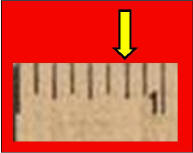Looking at the picture left and counting from the start or zero mark there are 6 spaces or 6/8 of an inch.  Dividing 8 by 6 produces 1.333 which is NOT a whole number (one with no decimal value left over.)  However  both numbers can be made smaller.  Any number or Fraction can be multiplied or divided by 1 without changing its value.  This can be accomplished by multiplying or dividing both the Numerator and Denominator by the same number.  Both the Numerator and Denominator could be multiplied by 2 (actually 2/2) that would give 6 * 2= 12 for a Numerator and 8 * 2 or 16 for the Denominator.  The Fraction would become 12/16; this is the identical value as 6/8.  That would be accurate and a person could be told to say "cut  a steel rod  12/16 of an inch long."   However the objective is to have the smallest numbers.  Both Numerator and Denominator could be divided by 2.  That would give 6/2 = 3 for a Numerator and 8/2=4 for a Denominator.  The Fraction now becomes 3/4.  It is the same value as 6/8 but with smaller numbers.  Notice on the ruler some lines are longer than others.  In this case the longest lines are quarters of an inch rather than eighths.  Three of these longest  (quarter) lines could have been counted and the proper value found more quickly as the third quarter or the 3/4 mark!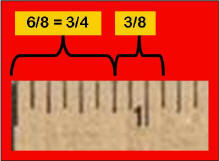If two Fraction dimensions are to be added (or subtracted) the numbers in the Denominator (bottom numbers) must be the same.  Suppose two items are to be measured and a total length presented.  If one is 3/4 inches and the other 3/8 inches what is the total length?  Quarters and eights can not be added directly.  It is best to convert all measurements to the largest Denominator, 8ths in this case.  Therefore 3/4 becomes 6/8 (remember the Numerator and Denominator can always be multiplied by the same number which is actually multiplying the Fraction by 1.)  The addition is then with 6/8 + 3/8.  The picture left shows the answer graphically.  But let’s get the answer using math.  To add Fractions, 6 of the eight parts are being added to 3 of the eight parts.  So only add the Numerator values (top numbers.)  Therefore 6 + 3 = 9 or 9 eights. Stated as a Fraction, 9/8.  Whenever the Numerator is bigger than the Denominator the Numerator can be divided by the Denominator to change to a whole number and possibly a  Fraction remainder.  In this case, 8 goes into 9 once with one left over or 8/8 + 1/8 or 1 1/8 inches.  This mark can be seen on the ruler as one space after 1 inch.  There would be nothing wrong with saying the answer was 9/8 but suppose the result was 13 inches and 3/8 inches.  It could be stated as 13 * 8 or 104/8 + 3/8 or 104 + 3 =107/8.  If it was necessary to measure using that dimension,  104  would be a lot of 1/8’s for someone to count!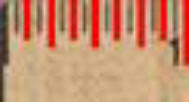A 1 inch ruler mark is often split into 16 segments.   These smaller marks are still easy to read (compared to 32 marks which are used for some steel rulers.)  The picture left shows this segmentation with the 1/8 inch marks and the 1/4 inch marks shown as longer red lines.  The 1/16 inch marks, shown in black, are the shortest.   This makes it easier to read quickly.

A tape measure is the same as a ruler except it is longer and flexible.  There are also some interesting things to note about most metal tapes. The picture left is a magnified section of a ruler.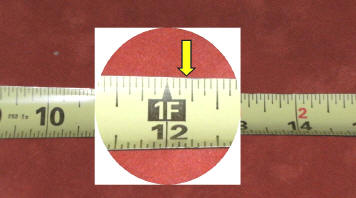This ruler is segmented into 1/16ths or 16 segments in an inch.  It is also labeled in feet as well as inches.  If a measurement is to be made to the yellow arrow it is located at the 12 inch or 1 foot mark plus 3 spaces.  therefore there are 12 inches and 3/16 inches from the end of the ruler to the yellow mark. The mark is said to be 12  and 3/16 inches or 1 foot 3/16 inches-both would be correct.There are a few other things that should be understood about a tape measure:

As seen in the picture right there is a metal tab at the start end of the tape.  It is usually attached with two rivets.  However it is loose.  Do Not tighten these rivets with a hammer!   The tab is  loose for a reason.  I have talked with folks who think the ruler was made wrong and the rivet was just not tight!  That is wrong. It has a very useful purpose and provides more accurate readings if used properly.  It takes care of the thickness of the metal tab when making outside and inside measurements.  We'll explain.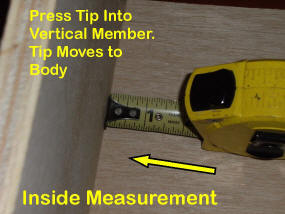If you’re making what is known as an “inside” measurement (picture left) - after  the tape is locked in position (with the black button on top) - the tape is pushed with a slight force into the part to be measured.  The looseness in the tab is designed to take care of the thickness of the metal tab "IF" it is pushed slightly to the vertical item being measured.  The tab moves in toward the ruler and provides an accurate measurement by properly positioning the zero location.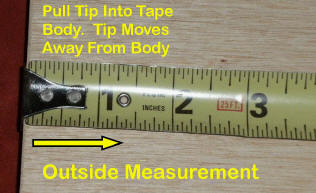In the photo right, the tape is being used to measure from the "outside" edge of an item.

The tape is pulled slightly away from the edge being measured and the tab moves slightly away from the tape measure body.  It moves the thickness of the tab so that the zero point is now on the inside or from the photo the right side of the tab.  This places the zero location as necessary to have it measure properly.  This movement is accounting for the thickness of the metal tab.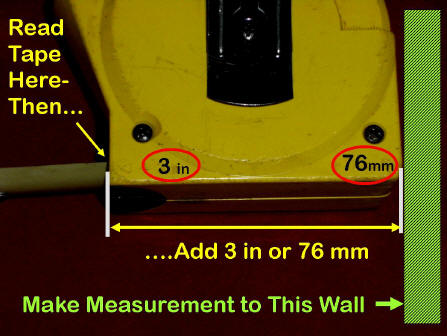The tape measure body often has a number printed on the base.  This is a value that can be used when making an inside measurement.

The measured value is read where the tape enters the tape body and the value of its width added to that reading.  For this ruler it is 3 inches.  The number 3  is added to the measured value to get the total measurement.

Metric: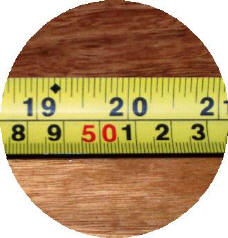Metric Units (now called SI for international Standard) are used by about every country accept the US.  Many items such as cars and trucks made and purchased in the US today use SI dimensions, bolts etc!  since parts are made all over the world, many industries have standardized on metric or SI  units

Metric is actually a simpler system and does not use Fractions!  All units are in 10ths.  The basic length measure is the meter (slightly longer that our yard.)  The preferred unit smaller than a meter is a 1000th of a meter called a millimeter (the abbreviation is mm.)  100rdth of a meter is called a centimeter (abbreviated as cm).  A cm = 10 mm's.  Everything is expressed in 10ths or multiples of 10.  The ruler in the picture shows inches on top and metric on the bottom.  The top is segmented in 1/16th of an inch.  The metric is labeled centimeters with segments in millimeters.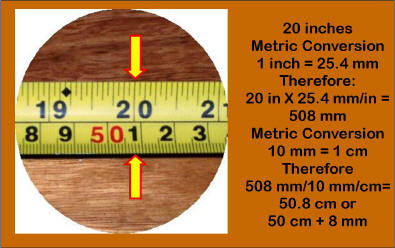The picture left shows a conversion from 20 inches to metric.  The ruler has both US Units and metric units. As noted, 20 inches must be multiplied by the conversion factor of 1 inch = 25.4 mm.  Therefore 20 inches = 508 mm.  Then 508 mm divided by 10 mm/cm = 50.8.  Note all we have to do to divide by 10 is move the decimal over 1 place.  That yields 50.8.  Or 50 cm and 0.8 cm.  But a cm is 10 mm.  So it could be written as 50.8 cm or 50 cm + 8 mm.

Below are some Common Welding Metric Conversions

 COMMON METRIC WELDING CONVERSIONS TO CONVERT FROM MULTIPLY BY TO OBTAIN THIS METRIC TERM inch (in) 25.4 mm foot (ft) 305 mm foot (ft) 0.305 m inches per min travel (ipm) 0.42 mm/sec impact (ft-lb) 1.36 Joules pound (lb) 0.454 kg ft3/hr (CFH) 0.47 L/min pressure (psi) 6.9 kPa strength (psi) 0.0069 MPa area (ft2) 0.093 m2 volume (in3) 1640 mm3 heat input (J/in) 39.4 J/m Welding Math and Physics PDF There is Much More Welding Math (and some Science) in Our 87 Page Self Study Program, "Welding Math and Science; Part # WMS This program provides basic information to help welding students and welding supervisors understand welding process characteristics using basic math and science.  Includes and Appendix with Simplified Basic Math Functions and Metric Conversions.  CLICK for Purchase Page